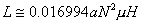# Brooks Coil and Calculator

A Brooks Coil is generally known as a special case of the circular coil inductor of rectangular cross section that is implemented to achieve the maximum inductance with a given length of wire. As can be seen from the picture below, the coil has a square cross section and the inner diameter is equal to twice the height (or width) of the coil winding.Diagram for a Brooks Coil, Maximum Inductance for a Given Length of Wire

The inductance for a Brooks coil can be found from the following equation:Equation for a Brooks Coil inductance using the coil width or height in cm

where c is the height and width of the coil winding (in cm) and N is the number of turns. A second formula is shown below (Grover, Frederick W., Inductance Calculations Working Formulas and Tables, D. Van Nostrand Company, 1946, p98. ISBN:0486495779):Equation for a Brooks Coil inductance using the coil mean radius in cm

where a is the mean radius of the inductor (in cm) and N is the number of turns.

The calculator below can be used to determine the inductance values for a specific Brooks coil configuration (Coil height/width and number of turns) using the first formula above. It will also determine the inner, outer, and mean radii based on that geometry. Credit for the initial Javascript code used in the calculator is given to Ray Allen who has a number of similar useful calculators on his website, Pulsed Power Portal.

Calculate Brooks Coil Parameters

 Parameter Value Units Inputs: Coil Height/Width centimetersmillimetersmicrometersnanometersmetersinchesfeetmilsyards____________________ N (# of Turns) Outputs: L (Inductance) micro-HenrysHenryskilo-Henrysmega-Henrysgiga-Henrysmilli-Henrysnano-Henrys____________________ Inner Radius centimetersmillimetersmicrometersnanometersmetersinchesfeetmilsyards____________________ Mean Radius centimetersmillimetersmicrometersnanometersmetersinchesfeetmilsyards____________________ Outer Radius centimetersmillimetersmicrometersnanometersmetersinchesfeetmilsyards____________________

Output Format:

 Select Format: Scientific Engineering Fixed

Send consulting inquiries, comments, and suggestions to richard.ness@nessengr.com